﻿ 基于压电复合纤维(MFC)的太阳翼帆板振动主动抑制

# 基于压电复合纤维(MFC)的太阳翼帆板振动主动抑制Active Vibration Suppression of Solar Array Based on MFC

Abstract: The energy capture devices used by spacecraft in space are basically solar array. Although the styles and structures are different, they are basically plate-shaped structures. The modal damping in space is small. If no measures are taken to suppress the vibration, the small amplitude vibration of these plate-shaped structures will last for a long time, which will affect the normal operation of spacecraft. In this paper, the active vibration suppression of solar array is studied. Firstly, the overall dynamic equation of the vibration suppression system of solar wing panel is determined by dynamic modeling, and the vibration modes of each order of the model are obtained by using HyperWorks. Then, the feedback control program is written by LabVIEW, and PID regulating parameters are set. Finally, the experimental model is built and the sensor/actuator is installed. The vibration curve is compared with the simulation, and the simulation control parameters are referred. Set the actual control parameters, and compare the vibration suppression effect of simulation and experiment. Through the comparison of simulation and experiment, it can be seen that the active vibration suppression system can effectively suppress the vibration of the sailboard, especially the micro amplitude vibration suppression effect is obvious.

1. 引言

2. 动力学建模

2.1. 薄板结构动力学建模

$m\stackrel{¨}{\delta }+k\delta ={F}_{1}$ (1)

$M\stackrel{¨}{\delta }+C\stackrel{˙}{\delta }+K\delta =F$ (2)

2.2. MFC作传感器方程

MFC根据正压电效应来俘获能量，并将其转换为电信号。帆板振动时，对于传感器MFC来说，受到交变的激励使其变形，则MFC将产生交变的电荷。MFC压电复合纤维满足电学短路、机械自由的条件，故采用第一类的压电方程研究其输出信号与各因素之间的关系，压电方程为：

$\begin{array}{l}{D}_{m}={d}_{mi}{\sigma }_{i}+{\epsilon }_{mn}^{T}{E}_{n}\\ \left(m,n=1,2,3:i=1,2,3,4,5,6\right)\end{array}$ (3)

${D}_{3}={d}_{31}{\sigma }_{1}$ (4)

$Q={d}_{31}{\sigma }_{1}S={d}_{31}E\epsilon S$ (5)

${V}_{MFC}=\frac{Q}{{C}_{MFC}}=\frac{{d}_{31}E\epsilon S}{{C}_{MFC}}$ (6)

2.3. MFC作致动器方程

MFC作致动器式时，根据逆压电效应，MFC产生的作动力 ${F}_{e}$ 可表示为：

${F}_{e}=-\alpha V$ (7)

$\alpha =\lambda {C}_{MFC}$ (8)

2.4. 整体模型的控制状态空间方程

$M\stackrel{¨}{\delta }+C\stackrel{˙}{\delta }+K\delta +\alpha V=F$ (9)

$\left\{\begin{array}{l}\stackrel{¨}{\delta }=-{M}^{-1}C\stackrel{˙}{\delta }-{M}^{-1}K\delta -{M}^{-1}\alpha V+{M}^{-1}F\\ {V}_{MFC}=\frac{{d}_{31}E\epsilon S}{{C}_{MFC}}\end{array}$ (10)

$\left\{\begin{array}{l}\stackrel{˙}{X}=AX+BU+{B}_{f}f\\ Y\left(t\right)\text{=}CX\end{array}$ (11)

3. 程序设计

3.1. 激励信号产生部分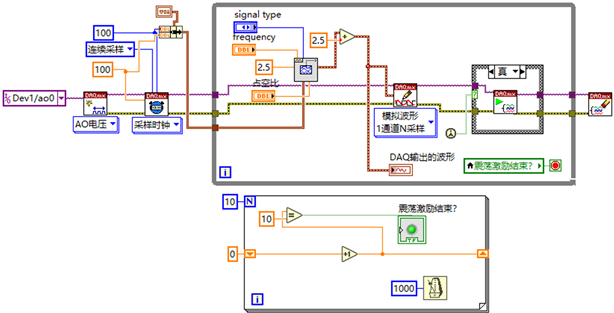Figure 1. Excitation signal generator

3.2. 反馈信号读取部分s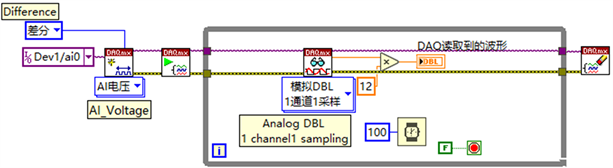3.3. 振动主动抑制算法部分

$u\left(t\right)={K}_{p}e\left(t\right)+{K}_{\text{i}}{\int }_{0}^{t}e\left(\tau \right)d\tau +{K}_{d}\frac{de\left(t\right)}{dt}$ (12)

$u\left(k\right)={K}_{p}\left[e\left(k\right)+\frac{T}{{T}_{i}}\underset{j=0}{\overset{k}{\sum }}e\left(j\right)+\frac{{T}_{d}}{T}\left[e\left(k\right)-e\left(k-1\right)\right]\right]$ (13)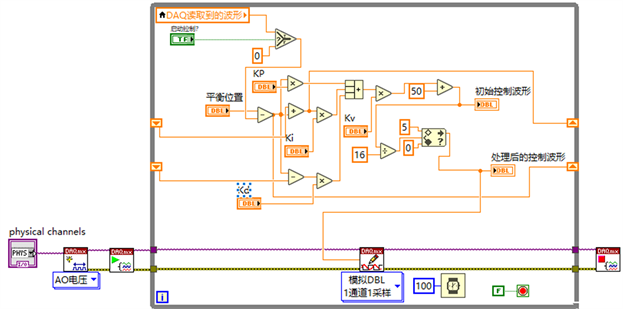Figure 3. PID control program

3.4. 数据保存程序Figure 4. Data saving program

4. 仿真与实验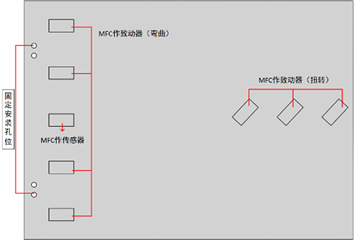(a)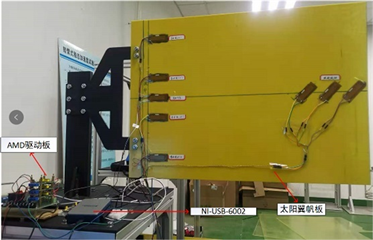(b)

Figure 5. The model of solar panel is compared with the real object. (a) Sailboard model; (b) Vibration suppression prototype system of sailboard

MFC采用SmartMateral公司代理生产的M4312-P1型，其材料特性和环氧树脂板的材料特性如下表1所示：Table 1. Material parameter table of M4312-P1 and experimental sailboard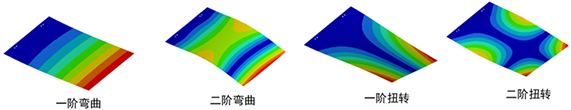Figure 6. The first four modes of modal analysis of sailboardTable 2. Analysis of the first four modes of the sailboard by Hyperworks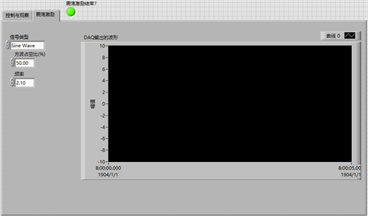(a)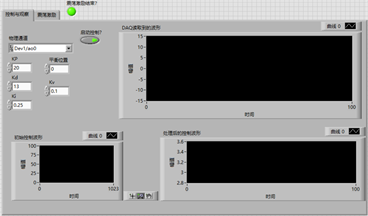(b)

Figure 7. Active vibration suppression front panel. (a) Front panel display excitation; (b) Front feedback control panel with PID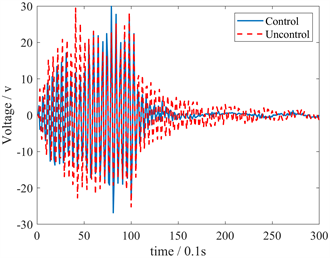Figure 8. Comparison of free vibration and active vibration suppression of sailboard

5. 总结

 欧进萍. 结构振动控制——主动、半主动和智能控制[M]. 北京: 科学出版社, 2003.

 张思马. 基于激光位移传感器测量的柔性板振动控制研究[D]: [硕士学位论文]. 华南理工大学, 2016.

 杜平安, 于亚婷, 刘建涛. 有限元法: 原理、建模及应用[M]. 北京: 国防工业出版社, 2011.

 Rivers, M.B. and Balakrishna, S. (2014) NASA Common Research Model Test Envelope Extension with Active Sting Damping at NTF. AIAA, 3135. https://doi.org/10.2514/6.2014-3135

 Thomson, M.W. (2002) AstroMesh Deployable Reflectors for Ku- and Ka-Band Commercial Satellites. 20th AIAA International Communication Satellite Systems Conference and Exhibit. https://doi.org/10.2514/6.2002-2032

 Alaimo, A., Milazzo, A. and Orlando, C. (2016) A Smart Composite-Piezoelectric One-Dimensional Finite Element Model for Vibration Damping Analysis. Journal of Intelligent Material Systems & Structures, 27, 1362-1375. https://doi.org/10.1177/1045389X15591380

 Kiran, R., Kumar, A., Kumar, R., et al. (2018) Poling Direction Driven Large Enhancement in Piezoelectric Performance. Scripta Materialia, 151, 76-81. https://doi.org/10.1016/j.scriptamat.2018.03.029

 Kiran, R., Kumar, A., Kumar, R., et al. (2019) Effect of Poling Direction and Porosity on Piezoelectric Figures of Merit: A Numerical Study. European Physical Journal Plus, 134, 103. https://doi.org/10.1140/epjp/i2019-12458-8

 Lezgy-Nazargah, M. (2016) Efficient Coupled Refined Finite Element for Dynamic Analysis of Sandwich Beams Containing Embedded Shear-Mode Piezoelectric Layers. Mechanics of Advanced Materials and Structures, 23, 337-352. https://doi.org/10.1080/15376494.2014.981617

 Sharma, S., Kumar, A., Kumar, R., Talha, M. and Vaish, R. (2020) Active vibration Control of Smart Structure Using Poling Tuned Piezoelectric Material. Journal of Intelligent Material Systems and Structures, 31, 1298-1313. https://doi.org/10.1177/1045389X20917456

 Kiran, R., Kumar, A., Kumar, R. and Vaish, R. (2018) Poling Direction Driven Large Enhancement in Piezoelectric Performance. Scripta Materialia, 151, 76-81. https://doi.org/10.1016/j.scriptamat.2018.03.029

 Zhu, Y., Zheng, X., Li, L., Yu, Y., Liu, X. and Chen, J. (2017) Evaluation of Shear Piezoelectric Coefficient d 15 of Piezoelectric Ceramics By Using Piezoelectric Cantilever Beam in Dynamic Resonance. Ferroelectrics, 520, 202-211. https://doi.org/10.1080/00150193.2017.1388838

 Chen, D., Carter, E. and Kamlah, M. (2016) Deformation Behavior of Lead Zirconate Titanate Ceramics under Uniaxial Compression Measured by the Digital Image Correlation Method. Smart Materials and Structures, 25, Article ID: 097001. https://doi.org/10.1088/0964-1726/25/9/097001

 欧进萍. 结构振动控制——主动、半主动和智能控制[M]. 北京: 科学出版社, 2003.

 张思马. 基于激光位移传感器测量的柔性板振动控制研究[D]: [硕士学位论文]. 华南理工大学, 2016.

 杜平安, 于亚婷, 刘建涛. 有限元法: 原理、建模及应用[M]. 北京: 国防工业出版社, 2011.

 Rivers, M.B. and Balakrishna, S. (2014) NASA Common Research Model Test Envelope Extension with Active Sting Damping at NTF. AIAA, 3135.
https://doi.org/10.2514/6.2014-3135

 Thomson, M.W. (2002) AstroMesh Deployable Reflectors for Ku- and Ka-Band Commercial Satellites. 20th AIAA International Communication Satellite Systems Conference and Exhibit. https://doi.org/10.2514/6.2002-2032

 Alaimo, A., Milazzo, A. and Orlando, C. (2016) A Smart Composite-Piezoelectric One-Dimensional Finite Element Model for Vibration Damping Analysis. Journal of Intelligent Material Systems & Structures, 27, 1362-1375.
https://doi.org/10.1177/1045389X15591380

 Kiran, R., Kumar, A., Kumar, R., et al. (2018) Poling Direction Driven Large Enhancement in Piezoelectric Performance. Scripta Materialia, 151, 76-81. https://doi.org/10.1016/j.scriptamat.2018.03.029

 Kiran, R., Kumar, A., Kumar, R., et al. (2019) Effect of Poling Direction and Porosity on Piezoelectric Figures of Merit: A Numerical Study. European Physical Journal Plus, 134, 103. https://doi.org/10.1140/epjp/i2019-12458-

 Lezgy-Nazargah, M. (2016) Efficient Coupled Refined Finite Element for Dynamic Analysis of Sandwich Beams Containing Embedded Shear-Mode Piezoelectric Layers. Mechanics of Advanced Materials and Structures, 23, 337-352.
https://doi.org/10.1080/15376494.2014.981617

 Sharma, S., Kumar, A., Kumar, R., Talha, M. and Vaish, R. (2020) Active vibration Control of Smart Structure Using Poling Tuned Piezoelectric Material. Journal of Intelligent Material Systems and Structures, 31, 1298-1313.
https://doi.org/10.1177/1045389X20917456

 Kiran, R., Kumar, A., Kumar, R. and Vaish, R. (2018) Poling Direction Driven Large Enhancement in Piezoelectric Performance. Scripta Materialia, 151, 76-81. https://doi.org/10.1016/j.scriptamat.2018.03.029

 Zhu, Y., Zheng, X., Li, L., Yu, Y., Liu, X. and Chen, J. (2017) Evaluation of Shear Piezoelectric Coefficient d 15 of Piezoelectric Ceramics By Using Piezoelectric Cantilever Beam in Dynamic Resonance. Ferroelectrics, 520, 202-211.
https://doi.org/10.1080/00150193.2017.1388838

 Chen, D., Carter, E. and Kamlah, M. (2016) Deformation Behavior of Lead Zirconate Titanate Ceramics under Uniaxial Compression Measured by the Digital Image Correlation Method. Smart Materials and Structures, 25, Article ID: 097001.
https://doi.org/10.1088/0964-1726/25/9/097001

Top